every-thing.net determines the number of possibilities of everything:
every thought, every person, every society, every object, and every history.

Using the 2 possible states of a neuron, firing or not firing, in combination
with the number of neurons in the human brain, every-thought determines
the number of possible thoughts. By combining the ova of all fertile females
on the planet with the available human sperm, every-person determines the
number of possible persons that can be created. Using a world population
of 6240272424 persons, every-society is determined, ranging in
membership from 2 to 6240272424 persons. Using the average size of
an atom, the number of different atoms and the size of the universe,
every-object determines, the number of possible structures of the universe,
each structure containing all matter within the universe. A data file format
is also proposed which contains a digital representation of the molecular
structure of the universe. By viewing history as the transformation of the
universe’s molecular structure from one moment to the next, every-history
determines the number of possible histories which could have happened
over the 13500000000 years of available time
.

Complete calculations for every-thing.net would require over 4.28 googol
octillion (4.28 x 10^127) years of computer processing, a duration of time
31700000000000000000000000000000000000000000000000000000000
00000000000000000000000000000000000000000000000000000000000
times greater than the history of the universe.

Single numbers--up to 240 pages in length--are available to users under the
yellow arrow icons, although these numbers may represent as little as
0.00000000000000000000000000000000000000000000000000000000000
000000000000000000000000000000000000000000000000000000000000
00000000003% of final calculations. Accurate calculations were obtained
through statistics, mathematics, permutations and programming software.
The Perl code used to create the calculations is provided here.

Certain large numbers are expressed using exponential notation. 3 x 10^9 is
read as 3 times 10 to the power of 9, equivalent to 3000000000.

PERMUTATIONS

 c = number of cells available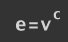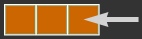`v = number of variations in a cell` e = every possible permutation

 EXAMPLE given 6 balls, 3 red and 3 green, the number of possible arrangements of 3 balls on 3 locations is determined below:permutation 1permutation 2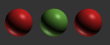permutation 3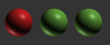permutation 4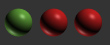permutation 5permutation 6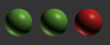permutation 7permutation 8

 number of possibilities can be determined using e = v^c different variations of balls available (red or green) = v = 2 different locations (cells) available = c = 3 total possible arrangements = e = 2^3 = 2 x 2 x 2 = 8

CALCULATION TIMES*

 ITEM PROCESSING TIME REQUIRED every-thought44.5 years every-person.001 second every-society4.04 years every-object148000000000000000000000000000000000000000000000000000000000000000000000000000000000000000000000000 years every-history42800000000000000000000000000000000000000000000000000000000000000000000000000000000000000000000000000000000000000000000000000000 years

* calculation times listed aboved were determined when everything.net was created in 2003 using a 400MHz G4 Mac operating at 1.35 GFLOPS

CALCULATION TIMES USING WORLDS FASTEST COMPUTER IN 2012*

 ITEM PROCESSING TIME REQUIRED every-thought3.11 minutes every-person.0000000000133 seconds every-society16.97 seconds every-object19714000000000000000000000000000000000000000000000000000000000000000000000000000000000000000 years every-history5701200000000000000000000000000000000000000000000000000000000000000000000000000000000000000000000000000000000000000000000 years

* Fujitsu K computer operating at 10.51 PLOPS, 7507143 times faster that the computer used in the initial 2003 calculations.

every-thing.net Don Ritter 2003